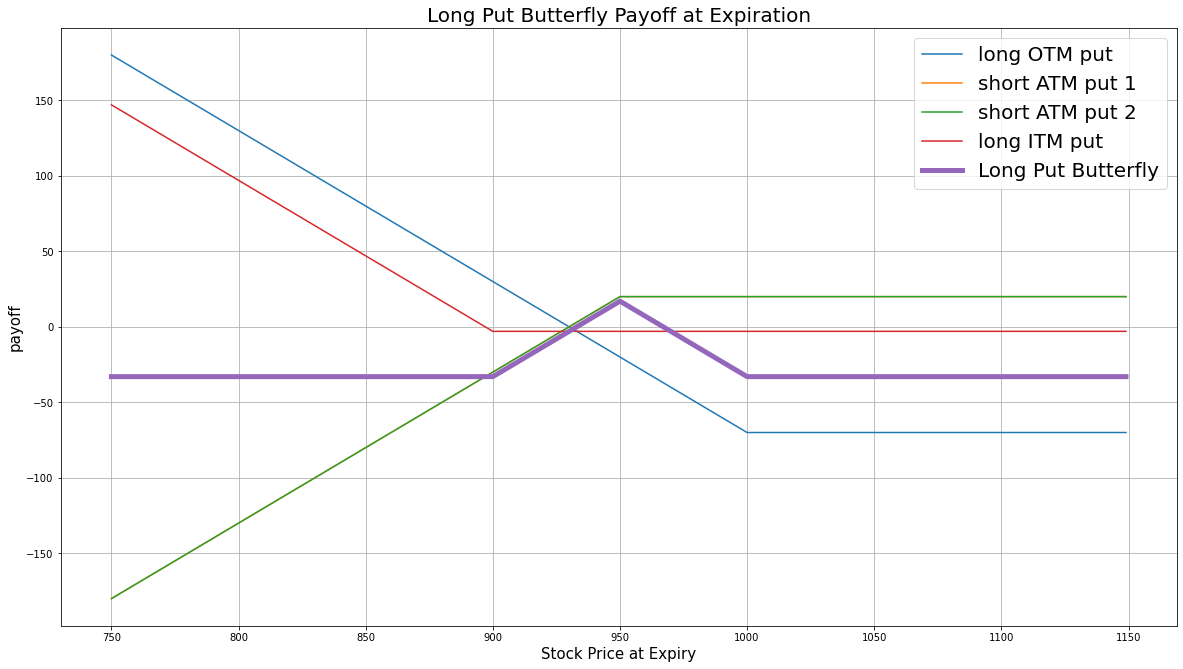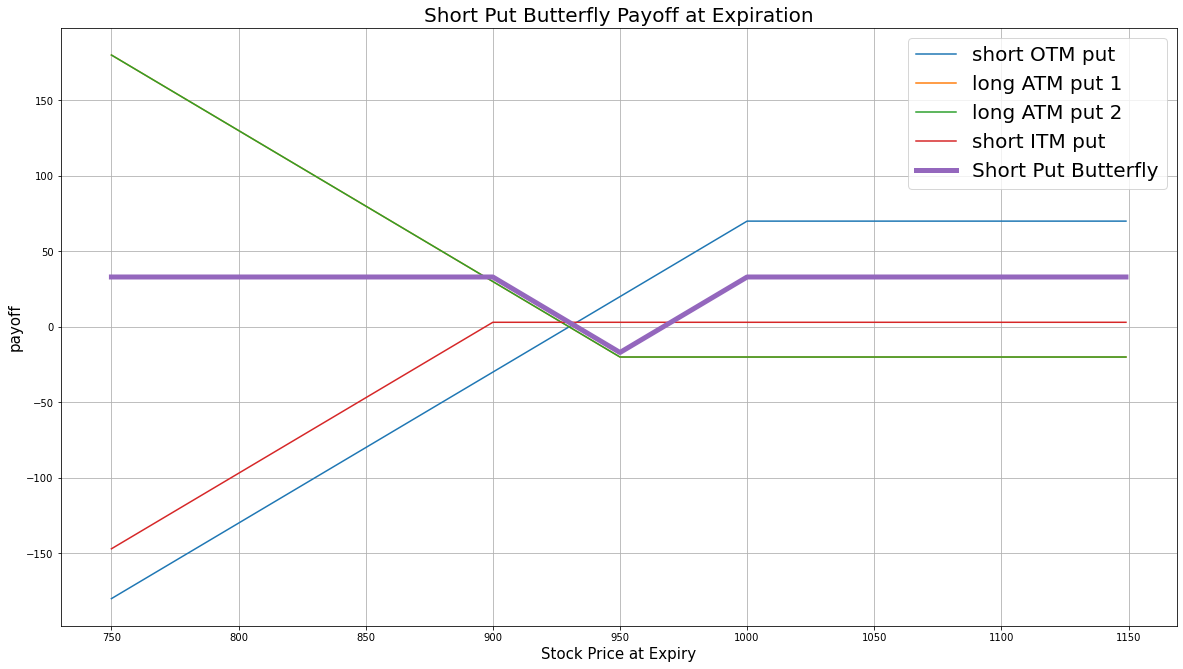# Option Strategies

## Put Butterfly

### Introduction

Put butterfly is the combination of a bull put spread and a bear put spread. In this strategy, all the puts have the same underlying stock, the same expiration date, and the strike price distance of ITM-ATM and OTM-ATM put pairs are the same. The put butterfly strategy can be long or short.

#### Long Put Butterfly

The long put butterfly strategy consists of buying an ITM put, buying an OTM put, and selling 2 ATM puts. This strategy profits from low volatility.

#### Short Put Butterfly

The short put butterfly strategy consists of selling an ITM put, selling an OTM put, and buying 2 ATM puts. This strategy profits from a drastic change in underlying price.

### Implementation

Follow these steps to implement the put butterfly strategy:

1. In the Initialize method, set the start date, end date, cash, and Option universe.
2. private Symbol _symbol;

public override void Initialize()
{
SetStartDate(2017, 2, 1);
SetEndDate(2017, 3, 5);
SetCash(500000);

_symbol = option.Symbol;
option.SetFilter(universe => universe.IncludeWeeklys()
.Strikes(-15, 15)
.Expiration(TimeSpan.FromDays(0), TimeSpan.FromDays(31)));
}
def Initialize(self) -> None:
self.SetStartDate(2017, 2, 1)
self.SetEndDate(2017, 3, 5)
self.SetCash(500000)

self.symbol = option.Symbol
option.SetFilter(self.UniverseFunc)

def UniverseFunc(self, universe: OptionFilterUniverse) -> OptionFilterUniverse:
return universe.IncludeWeeklys().Strikes(-15, 15).Expiration(timedelta(0), timedelta(31))
3. In the OnData method, select strikes and expiration date of the contracts in the strategy legs.
4. public override void OnData(Slice slice)
{
if (Portfolio.Invested) return;

// Get the OptionChain
var chain = slice.OptionChains.get(_symbol, null);
if (chain == null || chain.Count() == 0) return;

// Select an expiry date
var expiry = chain.OrderByDescending(x => x.Expiry).First().Expiry;

// Select the put contracts that expire on the selected date
var puts = chain.Where(x => x.Expiry == expiry && x.Right == OptionRight.Put);
if (puts.Count() == 0) return;

// Sort the put contracts by their strike prices
var putStrikes = puts.Select(x => x.Strike).OrderBy(x => x);

// Get the ATM strike price
var atmStrike = puts.OrderBy(x => Math.Abs(x.Strike - chain.Underlying.Price)).First().Strike;

// Get the distance between lowest strike price and ATM strike, and highest strike price and ATM strike
// Get the lower value as the spread distance as equidistance is needed for both sides
var spread = Math.Min(Math.Abs(putStrikes.First() - atmStrike), Math.Abs(putStrikes.Last() - atmStrike));

// Select the strike prices of the strategy legs
var itmStrike = atmStrike + spread;
var otmStrike = atmStrike - spread;
def OnData(self, slice: Slice) -> None:
if self.Portfolio.Invested: return

# Get the OptionChain
chain = slice.OptionChains.get(self.symbol, None)
if not chain: return

# Select an expiry date
expiry = sorted(chain, key = lambda x: x.Expiry, reverse=True).Expiry

# Select the put contracts that expire on the selected date
puts = [i for i in chain if i.Expiry == expiry and i.Right == OptionRight.Put]
if len(puts) == 0: return

# Sort the put contracts by their strike prices
put_strikes = sorted([x.Strike for x in puts])

# Get the ATM strike price
atm_strike = sorted(puts, key=lambda x: abs(x.Strike - chain.Underlying.Price)).Strike

# Get the distance between lowest strike price and ATM strike, and highest strike price and ATM strike.
# Get the lower value as the spread distance as equidistance is needed for both sides
spread = min(abs(put_strikes - atm_strike), abs(put_strikes[-1] - atm_strike))

# Select the strike prices of the strategy legs
otm_strike = atm_strike - spread
5. In the OnData method, call the OptionStrategies.PutButterfly method and then submit the order.
6. var optionStrategy = OptionStrategies.PutButterfly(_symbol, itmStrike, atmStrike, otmStrike, expiry);
Buy(optionStrategy, 1);    // if long put butterfly
Sell(optionStrategy, 1);   // if short put butterfly
option_strategy = OptionStrategies.PutButterfly(self.symbol, itm_strike, atm_strike, otm_strike, expiry)
self.Buy(option_strategy, 1)    # if long put butterfly
self.Sell(option_strategy, 1)   # if short put butterfly

Option strategies synchronously execute by default. To asynchronously execute Option strategies, set the asynchronous argument to Falsefalse. You can also provide a tag and order properties to the Buy and Sell methods.

Buy(optionStrategy, quantity, asynchronous, tag, orderProperties);
Sell(optionStrategy, quantity, asynchronous, tag, orderProperties);

self.Buy(option_strategy, quantity, asynchronous, tag, order_properties)
self.Sell(option_strategy, quantity, asynchronous, tag, order_properties)


### Strategy Payoff

The put butterfly can be long or short.

#### Long Put Butterfly

The long put butterfly is a limited-reward-limited-risk strategy. The payoff is

$$\begin{array}{rcll} P^{OTM}_T & = & (K^{OTM} - S_T)^{+}\\ P^{ITM}_T & = & (K^{ITM} - S_T)^{+}\\ P^{ATM}_T & = & (K^{ATM} - S_T)^{+}\\ P_T & = & (P^{OTM}_T + P^{ITM}_T - 2\times P^{ATM}_T + 2\times P^{ATM}_0 - P^{ITM}_0 - P^{OTM}_0)\times m - fee \end{array}$$ $$\begin{array}{rcll} \textrm{where} & P^{OTM}_T & = & \textrm{OTM put value at time T}\\ & P^{ITM}_T & = & \textrm{ITM put value at time T}\\ & P^{ATM}_T & = & \textrm{ATM put value at time T}\\ & S_T & = & \textrm{Underlying asset price at time T}\\ & K^{OTM} & = & \textrm{OTM put strike price}\\ & K^{ITM} & = & \textrm{ITM put strike price}\\ & K^{ATM} & = & \textrm{ATM put strike price}\\ & P_T & = & \textrm{Payout total at time T}\\ & P^{ITM}_0 & = & \textrm{ITM put value at position opening (debit paid)}\\ & P^{OTM}_0 & = & \textrm{OTM put value at position opening (debit paid)}\\ & P^{ATM}_0 & = & \textrm{ATM put value at position opening (credit received)}\\ & m & = & \textrm{Contract multiplier}\\ & T & = & \textrm{Time of expiration} \end{array}$$

The following chart shows the payoff at expiration:The maximum profit is $K^{ATM} - K^{OTM} + 2\times P^{ATM}_0 - P^{ITM}_0 - P^{OTM}_0$. It occurs when the underlying price is the same at expiration as it was when you open the trade. In this case, the payout of the combined bull put and bear put spreads are at their maximum.

The maximum loss is the net debit paid, $2\times P^{ATM}_0 - P^{ITM}_0 - P^{OTM}_0$. It occurs when the underlying price is below the ITM strike price or above the OTM strike price at expiration.

If the Option is American Option, there is risk of early assignment on the sold contracts.

#### Short Put Butterfly

The short put butterfly is a limited-reward-limited-risk strategy. The payoff is

$$\begin{array}{rcll} P^{OTM}_T & = & (K^{OTM} - S_T)^{+}\\ P^{ITM}_T & = & (K^{ITM} - S_T)^{+}\\ P^{ATM}_T & = & (K^{ATM} - S_T)^{+}\\ P_T & = & (2\times P^{ATM}_T - P^{OTM}_T - P^{ITM}_T - 2\times P^{ATM}_0 + P^{ITM}_0 + P^{OTM}_0)\times m - fee \end{array}$$ $$\begin{array}{rcll} \textrm{where} & P^{OTM}_T & = & \textrm{OTM put value at time T}\\ & P^{ITM}_T & = & \textrm{ITM put value at time T}\\ & P^{ATM}_T & = & \textrm{ATM put value at time T}\\ & S_T & = & \textrm{Underlying asset price at time T}\\ & K^{OTM} & = & \textrm{OTM put strike price}\\ & K^{ITM} & = & \textrm{ITM put strike price}\\ & K^{ATM} & = & \textrm{ATM put strike price}\\ & P_T & = & \textrm{Payout total at time T}\\ & P^{ITM}_0 & = & \textrm{ITM put value at position opening (credit received)}\\ & P^{OTM}_0 & = & \textrm{OTM put value at position opening (credit received)}\\ & P^{ATM}_0 & = & \textrm{ATM put value at position opening (debit paid)}\\ & m & = & \textrm{Contract multiplier}\\ & T & = & \textrm{Time of expiration} \end{array}$$

The following chart shows the payoff at expiration:The maximum profit is the net credit received, $P^{ITM}_0 + P^{OTM}_0 - 2\times P^{ATM}_0$. It occurs when the underlying price is below the ITM strike or above the OTM strike at expiration.

The maximum loss is $K^{ATM} - K^{OTM} - 2\times P^{ATM}_0 + P^{ITM}_0 + P^{OTM}_0$. It occurs when the underlying price at expiration is at the same price as when you opened the trade.

If the Option is American Option, there is risk of early assignment on the sold contracts.

### Example

The following table shows the price details of the assets in the long put butterfly algorithm:

AssetPrice ($)Strike ($)
ITM put37.80832.50
ATM put14.70800.00
OTM put5.70767.50
Underlying Equity at expiration829.08-

Therefore, the payoff is

$$\begin{array}{rcll} P^{OTM}_T & = & (K^{OTM} - S_T)^{+}\\ & = & (829.08-832.50)^{+}\\ & = & 0\\ P^{ITM}_T & = & (K^{ITM} - S_T)^{+}\\ & = & (829.08-767.50)^{+}\\ & = & 61.58\\ P^{ATM}_T & = & (K^{ATM} - S_T)^{+}\\ & = & (829.08-800.00)^{+}\\ & = & 29.08\\ P_T & = & (P^{OTM}_T + P^{ITM}_T - 2\times P^{ATM}_T + 2\times P^{ATM}_0 - P^{ITM}_0 - P^{OTM}_0)\times m - fee\\ & = & (61.58+0-29.08\times2-5.70-37.80+14.70\times2)\times100-1.00\times4\\ & = & -1072 \end{array}$$

So, the strategy losses \$1,072.

The following algorithm implements a long put butterfly Option strategy:

You can also see our Videos. You can also get in touch with us via Discord.